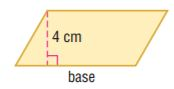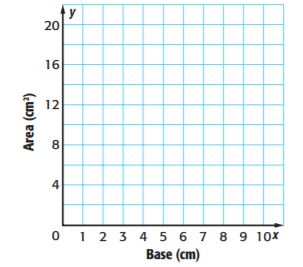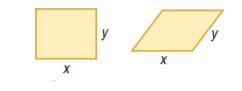Homework Explained - Math Practice 101Dear guest, you are not a registered member. As a guest, you only have read-only access to our books, tests and other practice materials.

As a registered member you can:

Registration is free and doesn't require any type of payment information. Click here to Register.
Go to page:
Chapter 9:Area;Lesson 1: Area of Parallelograms

Multiple Representations Draw five parallelograms that each have a height of 4 centimeters and different base measurements on centimeter grid paper.• Question 9

a. Table Make a table with a column for base, height, and area.

• Question 9

b. Graph Graph the ordered pairs (base, areal).• Question 9

c. Words Describe the graph.

### H.O.T ProblemsHigher Order Thinking

• Question 10

Persevere with Problems if x = 5 and y< x, which figure has the greater area? Explain your reasoning.• Question 11

Reason Inductively Explain how the formula for the area of a parallelogram is related to the formula for the area of a rectangle.

• Question 12

Reason Inductively Give an example of a triangle and a parallelogram that have the same area. Describe the bases and heights of each figure.Then state the area.

Yes, email page to my online tutor. (if you didn't add a tutor yet, you can add one here)